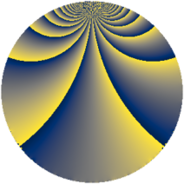# Properties

 Label 228.2.cLevel $228$ Weight $2$ Character orbit 228.c Rep. character $\chi_{228}(191,\cdot)$ Character field $\Q$ Dimension $36$ Newform subspaces $1$ Sturm bound $80$ Trace bound $0$

# Learn more

## Defining parameters

 Level: $$N$$ $$=$$ $$228 = 2^{2} \cdot 3 \cdot 19$$ Weight: $$k$$ $$=$$ $$2$$ Character orbit: $$[\chi]$$ $$=$$ 228.c (of order $$2$$ and degree $$1$$) Character conductor: $$\operatorname{cond}(\chi)$$ $$=$$ $$12$$ Character field: $$\Q$$ Newform subspaces: $$1$$ Sturm bound: $$80$$ Trace bound: $$0$$

## Dimensions

The following table gives the dimensions of various subspaces of $$M_{2}(228, [\chi])$$.

Total New Old
Modular forms 44 36 8
Cusp forms 36 36 0
Eisenstein series 8 0 8

## Trace form

 $$36q - 6q^{6} + O(q^{10})$$ $$36q - 6q^{6} + 8q^{10} + 4q^{12} - 8q^{16} + 16q^{18} + 8q^{21} - 12q^{22} - 2q^{24} - 28q^{25} + 12q^{28} - 12q^{30} - 28q^{34} - 22q^{36} - 16q^{37} - 12q^{40} + 10q^{42} + 16q^{45} - 4q^{46} + 32q^{48} - 44q^{49} - 36q^{52} - 20q^{54} - 4q^{58} - 4q^{60} + 16q^{61} + 24q^{64} + 24q^{66} - 16q^{69} + 36q^{70} - 36q^{72} - 8q^{73} - 32q^{78} - 40q^{81} + 72q^{82} - 20q^{84} + 16q^{85} - 16q^{88} - 56q^{90} + 8q^{93} - 56q^{94} + 2q^{96} + 40q^{97} + O(q^{100})$$

## Decomposition of $$S_{2}^{\mathrm{new}}(228, [\chi])$$ into newform subspaces

Label Dim. $$A$$ Field CM Traces $q$-expansion
$$a_2$$ $$a_3$$ $$a_5$$ $$a_7$$
228.2.c.a $$36$$ $$1.821$$ None $$0$$ $$0$$ $$0$$ $$0$$